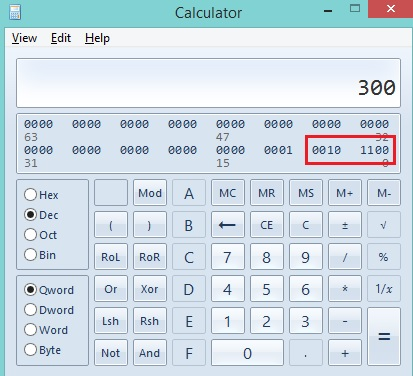# ‘unsigned char’ for memory optimization in c programming

Developers generally use int to store integer values, without thinking about data range, if the data range is less, we should use unsigned char.

## unsigned char

A type of char data type, unsigned char can store values between 0 to 255, so we can use unsigned char instead of short or int.

Here is an example:

```#include <stdio.h>
int main()
{
unsigned char value=0;

printf("Value is: %d\n",value);

value=50;
printf("Value is: %d\n",value);

value=255;
printf("Value is: %d\n",value);

return 0;
}
```

Output

```Value is: 0
Value is: 50
Value is: 255
```

Here we can see clearly that unsigned char is able to store values from 0 to 255.

## What will happen, if the value is greater than 255?

```int main()
{
unsigned char value=300;
printf("Value is: %d\n",value);
return 0;
}
```

Output

```value is: 44
```

Here the vale will be 44

### Why?

unsigned char store only 8 bits data into the memory, when the value is greater than only first 8 bits will be stored, see the given image.In this image 8 bits value is: 0010 1100 which is equivalent to 44.

### If the data value if negative?

We can use char instead of unsigned char to store data value between -128 to 127, char stores only 7 bits of the data, 8th bits is used for sign representation.

Here is an example:

```#include <stdio.h>
int main()
{
char value=0;

printf("Value is: %d\n",value);

value=-128;
printf("Value is: %d\n",value);

value=127;
printf("Value is: %d\n",value);

return 0;
}
```

Output

```Value is: 0
Value is: -128
Value is: 127
```